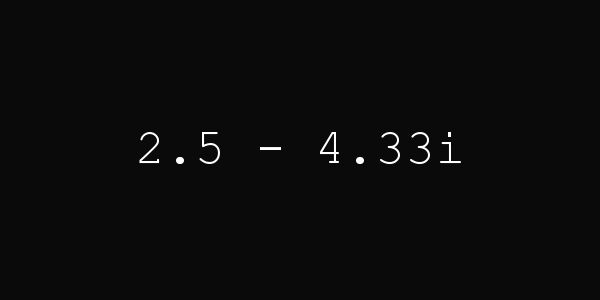# JClock VIII

2016-07-30, post № 135

programming, Pygame, Python, #clock, #complex number, #hour, #minute, #plane, #time

Interpreting the hour hand on a clock as a two-dimensional object on a plane, the hand’s tip can be seen as a complex number.
This clock converts the hour hand’s position into a complex number, sets the number’s length to the current minutes and displays it in the form$a+b\cdot i$.
The angle 𝜑 is determined by the hours passed ($\frac{2\cdot\pi\cdot\text{hour}}{12}=\frac{\pi\cdot\text{hour}}{6}$) but has to be slightly modified because a complex number starts at the horizontal axis and turns anti-clockwise whilst an hour hand starts at the vertical axis and turns — as the name implies — clockwise. Thus,$\varphi=(2\cdot\pi-\frac{\pi\cdot\text{hour}}{6})+\frac{\pi}{2}=(\frac{15-\text{hour}}{6})\cdot\pi$.

The complex number’s length is simply determined by the minutes passed. Because the length must not be equal to 𝟢, I simply add 𝟣:$|z|=k=\text{minute}+1$.
Lastly, to convert a complex number of the form$k\cdot e^{\varphi\cdot i}$ into the form$a+b\cdot i$, I use the formula$k\cdot(\cos{\varphi}+\sin{\varphi}\cdot i)=a+b\cdot i$.Source code: jclock-viii.py

# Weekday

2016-07-23, post № 134

programming, Python, #calculation, #date, #day, #month, #time, #year

Determining the weekday based on a date composed of day, month and year.
The program counts up all the days from the 1st of January 1 to the given date, divides it by 𝟩, looks at the remainder and returns the weekday.

Source code: weekday.py

# Triangular Squares

2016-07-16, post № 133

programming, Python, Wolfram Language, #equation, #number, #number theory, #numbers, #OEIS, #square, #triangle, #triangles

In a recent video, Matt Parker showed a triangular number that also is a square number, 𝟨, and asked if there were more.

A triangular number has the form$\frac{n^2+n}{2}$ — shown by Euler — and a square number has the form$m^2$.
Triangular squares are those numbers for which$\frac{n^2+n}{2}=m^2$ with$n,m\in\mathbb{N}$.
Examples are$\{0,1,6,35,204,1189,6930,\dots\}$ (sequence A001109 in OEIS).

To check if triangular numbers are square numbers is easy (code listed below), but a mathematical function would be nicer.
The first thing I tried was to define the triangular number’s square root as a whole number,$\sqrt{\frac{n^2+n}{2}}=\lfloor\sqrt{\frac{n^2+n}{2}}\rfloor$. This function does not return the square numbers that are triangular but the triangular numbers that are square.
The resulting sequence is$\{0,1,8,49,288,1681,9800,\dots\}$ (sequence A001108 in OEIS).

Source code: triangular-squares.py
Jonathan Frech's blog; built 2021/04/16 21:21:49 CEST